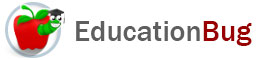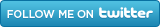PUBLIC SCHOOLS PRIVATE SCHOOLS SCHOOL DISTRICTS COLLEGES PUBLIC LIBRARIES JOBS BLOG RESOURCES
Career Education
Education Choices
Education Costs
Education History and General Information
Education Issues
Education Resources
Family Involvement in Education
Students w Special Needs
Subject Information and Homework Help
Teaching and Learning
Testing
By Types of Schools
By Specific Audiences
GeneralDivision

Division is the inverse of multiplication. This article has information on several different types of division including horizontal division and long division. Also, tips on special division, remainders, decimals, and repeating decimals.

Division is one of the four basic arithmetic operations, along with addition, subtraction, and multiplication. It is the inverse operation for multiplication, which means that it reverses to operation of multiplication, so if you begin with two numbers, multiply them, and then divide the result by one of them, you will be back to where you started.

Division is usually the last of the four arithmetic functions to be taught. Even though all four operations are just about equivalent on a calculator - where you enter one number, an operation sign, another number and an equal sign to gain the answer for any of them - doing division by hand can require more complex types of thought than the other operations for several reasons. For more information about division, keep reading

The Nomenclature of Division

Division has three different elements: the dividend is the number thatâ€™s being divided; the divisor is the number of parts itâ€™s being divided into; the quotient is the answer. Letâ€™s look at an example and relate it to multiplication:

Multiplication

 a x b = c multiplicand operation multiplier product Division c Â¸ b = a dividend operation divisor quotient

As a help to remembering these, if you need to know them, notice that the number that you start with in each case has a name that ends with the same two letters: nd. The number that you are using to operate on the first number has a  name with a suffix that in English refers to something that acts: -er and -or. And the result of the operation, in each case, is the one word out of the three that begins with a different letter: p instead of m; q instead of d.

There is one other important word in division: factors. Factors are the potential divisors that result in an integer quotient (i.e., an answer that is a whole number). It is helpful to be able to identify the possible factors of a dividend.

Forms of Division

Division problems are presented in three main ways, besides as word problems that the reader has to formulate him- or herself into a division problem.

â€¢ Horizontal

Horizontal format is usually the first way students see division problems. Horizontal form is the way the division is presented in the chart above, but without the chart, but using the letters to represent the same elements:

c Ã· b = a

Unless the division problem is very easy and can be done either from memory, by quick recall of the multiplication table (see below), or by a bit of subtracting, it is likely to be put into another format to be worked out.

â€¢ Long Division

This is the typical format for doing division on paper, again, using the elements from the chart.

â€¢ Fraction Format

As mentioned in the article â€œFractions,â€  the format of fractions can also be considered in terms of division. In this format, the dividend is placed in the numerator and the divisor in the denominator, with either a horizontal or a slanted slash between them:

c/b = a                 c   = a

b

Special Thinking for Division

There are several elements of division that make it require more complex thinking than the other arithmetic operations. Hereâ€™s a brief explanation of each:

If you begin an addition, subtraction, or multiplication problem with integers (that is, whole numbers), your answer will also have whole numbers. It is true that in subtraction you could begin with positive numbers and end up with an answer that is a negative number. Nevertheless, they can be taught in a controlled way. Division problems can end up with answers that are not integers.

â€¢ Remainders or Decimals

In working addition, subtraction, and multiplication problems, the answer is the answer. But in some division problems, one has to decide whether itâ€™s appropriate to have a remainder or to keep dividing. That is, the form of the answer is partly up to the person doing the math. This makes division more complex.

â€¢ Repeating or Recurring Decimals

Sometimes the answer to a division problem can have a decimal that is unending. This is true, for example, if one divides 1 into 3 parts. There is no end to the answer. This situation requires the person doing the math to recognize the situation and make a decision about how to handle it, given the context. This, too, is more complex that situations one encounters in the other arithmetic operations.

Helps for Division

Three types of information can help you do division more flexibly when thereâ€™s a reason to do it without a calculator.

â€¢ Know the Multiplication Table